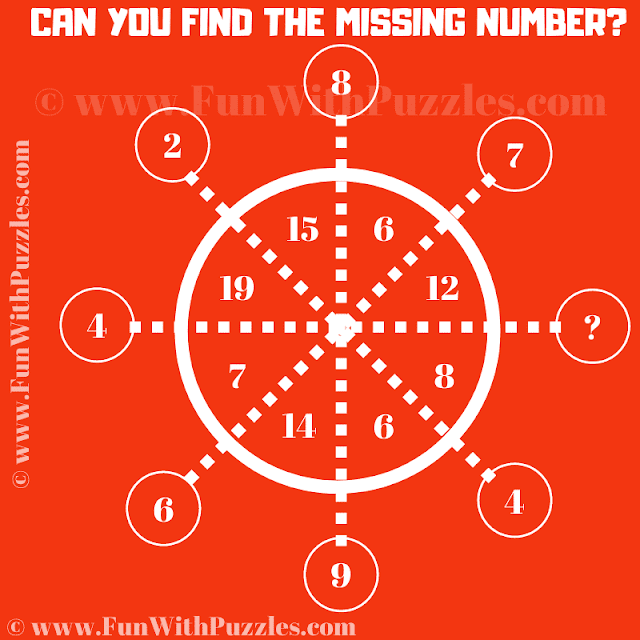This is a quick number puzzle for school students. Solving this puzzle will test your analytics ability and mathematical skills. In this number puzzle, you are shown some numbers inside and small and big circles. These numbers inside these circles relate to each other logically or mathematically. Your first challenge is to find this logical relationship among these numbers. Once you are able to crack this logical code, find the value of the missing number that will replace the question mark.
This is an easy quick number puzzle that one should be able to solve very quickly. Answer to this puzzle question is provided. However, if you could solve this puzzle without looking at the answer, write down your answer and logic in the comment box.Can you find the missing number?

The answer to this "Quick Number Puzzle", can be viewed by clicking on the answer button.